# NCERT Solutions for Class 10 Maths:Chapter 14 Statistics Exercise 14.1

In this page we have NCERT Solutions for Class 10 Maths:Chapter 14 Statistics for Exercise 14.1 on pages 270,271 and 272. Hope you like them and do not forget to like , social_share and comment at the end of the page.
Formula
The mean of grouped data can be obtained using
i.Direct method
$M= \frac { \sum f_i x_i}{\sum f_i}$
ii. assumed mean method
$M= a + \frac { \sum f_i d_i}{\sum f_i}$
iii. Step deviation method
$M= a + \frac { \sum f_i u_i}{\sum f_i} \times h$

## NCERT Solutions for Class 10 Maths Statistics Exercise 14.1

Question 1
A survey was conducted by a group of students as a part of their environment awareness programmes, in which they collected the following data regarding the number of plants in 20 houses in a locality. Find the mean number of plants per house.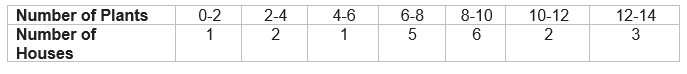Which method did you use for finding the mean, and why?
Solution
We will use direct method because the numerical value of fi and xi are small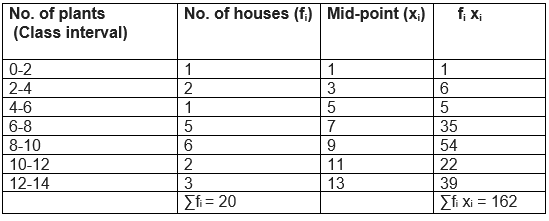$M= \frac { \sum f_i x_i}{\sum f_i}$ $Mean(M) = \frac {162}{20} = 8.1$

Question 2
Consider the following distribution of daily wages of 50 workers of a factory.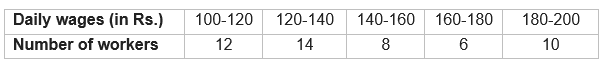Find the mean daily wages of the workers of the factory by using an appropriate method.
Solution
Here The value of mid-point (xi) is very large. So, assumed mean or step deviation method would be simple to calculate
Here the class interval is h = 20.
We can see that step deviation method would be best here
Now assumed mean A = 150 and class interval is h = 20.
So, u= (xi - A)/h
u= (xi - 150)/20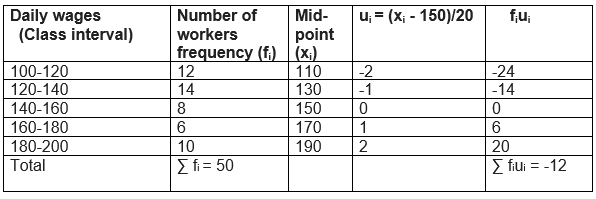$M= a + \frac { \sum f_i u_i}{\sum f_i} \times h$
=150 + (20 × -12/50) = 150 - 4.8 = 145.20
Thus, mean daily wage = Rs. 145.20
Question 3
The following distribution shows the daily pocket allowance of children of a locality. The mean pocket allowance is Rs 18. Find the missing frequency f.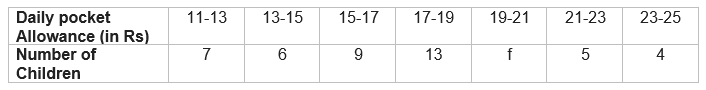Solution
Here, Mean= 18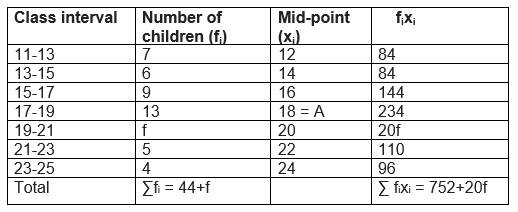$M= \frac { \sum f_i x_i}{\sum f_i}$
$18= \frac {(752+20f)}{(44+f)}$
$18(44+f) = (752+20f)$
40 = 2f
f = 20

Question 4
Thirty women were examined in a hospital by a doctor and the number of heart beats per minute were recorded and summarized as follows. Find the mean heart beats per minute for these women, choosing a suitable method.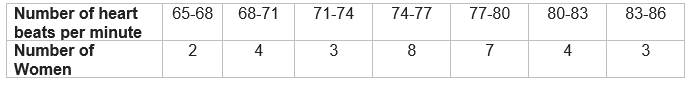Solution
x= (Upper limit + Lower limit)/2
Here The value of mid-point (xi) is very large. So, assumed mean or step deviation method would be simple to calculate
Now Class size (h) = 3
We can see that step deviation method would be best here
Now assumed mean A = 75.5 and class interval is h = 3
So, u= (xi - A)/h
u= (xi – 75.5)/3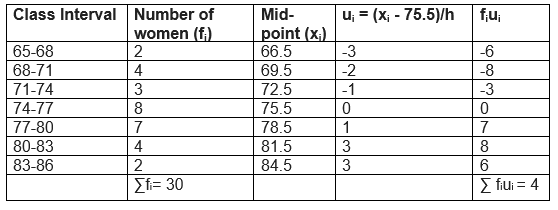$M= a + \frac { \sum f_i u_i}{\sum f_i} \times h$
= 75.5 + 3×(4/30) = 75.5 + 4/10 = 75.5 + 0.4 = 75.9
The mean heart beats per minute for these women is 75.9

Question 5
In a retail market, fruit vendors were selling mangoes kept in packing boxes. These boxes contained varying number of mangoes. The following was the distribution of mangoes per the number of boxes.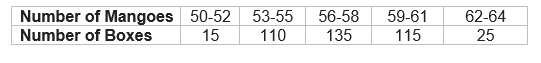Find the mean number of mangoes kept in a packing box. Which method of finding the mean did you choose?

Solution
Since, the given data is not continuous so we add 0.5 to the upper limit and subtract 0.5 from the lower limit.
Here, assumed mean (A) = 57
Class size (h) = 3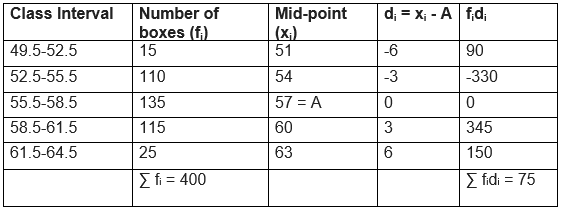$M= a + \frac { \sum f_i d_i}{\sum f_i}$
= 57 + (75/400) = 57 + 0.1875 = 57.19

Question 6
The table below shows the daily expenditure on food of 25 households in a locality.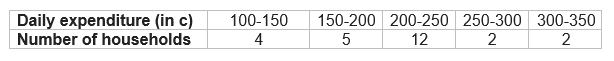Find the mean daily expenditure on food by a suitable method.

Solution
Here, assumed mean (A) = 225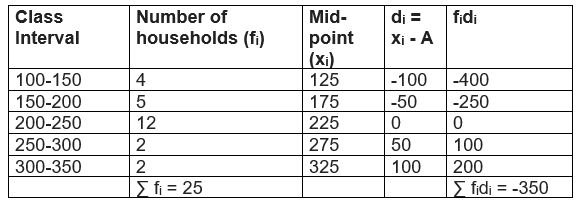$M= a + \frac { \sum f_i d_i}{\sum f_i}$
= 225 + (-350/25) = 225 - 14 = 211
The mean daily expenditure on food is 211

Question 7
To find out the concentration of SO2 in the air (in parts per million, i.e., ppm), the data was collected for 30 localities in a certain city and is presented below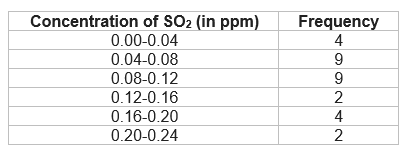Find the mean concentration of SO2 in the air.
Solution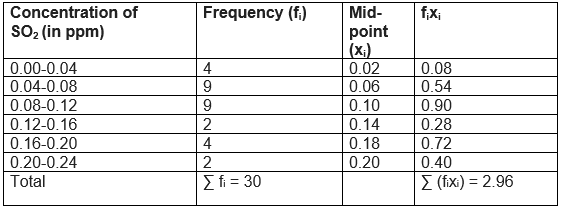$M= \frac { \sum f_i x_i}{\sum f_i}$
= 2.96/30 = 0.099 ppm

Question 8
A class teacher has the following absentee record of 40 students of a class for the whole
term. Find the mean number of days a student was absent.
 Number of days 0-6 6-10 10-14 14-20 20-28 28-38 38-40 Number of students 11 10 7 4 4 3 1

Solution
 Class interval Frequency (fi) Mid-point (xi) fixi 0-6 11 3 33 6-10 10 8 80 10-14 7 12 84 14-20 4 17 68 20-28 4 24 96 28-38 3 33 99 38-40 1 39 39 ∑ fi = 40 ∑ fixi = 499
$M= \frac { \sum f_i x_i}{\sum f_i}$
$M= \frac {499}{40} = 12.48$ days

Question 9.
The following table gives the literacy rate (in percentage) of 35 cities. Find the mean
literacy rate.

 Literacy rate (in %) 45-55 55-65 65-75 75-85 85-98 Number of cities 3 10 11 8 3

Solution
We will use here the step deviation method
Assumed mean =70
H=10

 Class Interval Frequency (fi) (xi) di = xi - a ui = di/h fiui 45-55 3 50 -20 -2 -6 55-65 10 60 -10 -1 -10 65-75 11 70 0 0 0 75-85 8 80 10 1 8 85-95 3 90 20 2 6 ∑ fi  = 35 ∑ fiui  = -2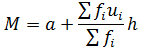= 70 + (-2/35) ? 10 = 69.42

## Summary

1. NCERT Solutions for Class 10 Maths:Chapter 14 Statistics Exercise 14.1 has been prepared by Expert with utmost care. If you find any mistake.Please do provide feedback on mail.You can download this as pdf
2. This chapter 13 has total 5 Exercise 14.1 and 14.2. This is the First exercise in the chapter.You can explore previous exercise of this chapter by clicking the link below

• Notes
• Assignments
• NCERT SolutionsGo back to Class 10 Main Page using below links

### Practice Question

Question 1 What is $1 - \sqrt {3}$ ?
A) Non terminating repeating
B) Non terminating non repeating
C) Terminating
D) None of the above
Question 2 The volume of the largest right circular cone that can be cut out from a cube of edge 4.2 cm is?
A) 19.4 cm3
B) 12 cm3
C) 78.6 cm3
D) 58.2 cm3
Question 3 The sum of the first three terms of an AP is 33. If the product of the first and the third term exceeds the second term by 29, the AP is ?
A) 2 ,21,11
B) 1,10,19
C) -1 ,8,17
D) 2 ,11,20